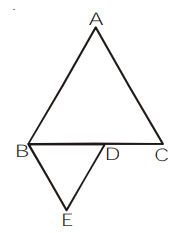# ABC and BDE are two equilateral triangles such that D is the mid-point of BC.

Question.

ABC and BDE are two equilateral triangles such that D is the mid-point of BC. Ratio of the areas of triangles ABC and BDE is

(1) 2 : 1

(2) 1 : 2

(3) 4 : 1

(4) 1 : 4

Solution:

$(\because B C=2 B D)$Since, both are equilateral triangles

$\Delta \mathrm{ABC} \sim \Delta \mathrm{EBD}$

$\frac{\operatorname{ar} \Delta \mathrm{ABC}}{\operatorname{ar} \Delta \mathrm{BDE}}=\left(\frac{\mathrm{BC}}{\mathrm{BD}}\right)^{2}=\left(\frac{2}{1}\right)^{2}=4: 1$